# Solutions of Electricity (Page No- 12 & 18) - Physics By Lakhmir Singh, Class 10 Class 10 Notes | EduRev

## Class 10 : Solutions of Electricity (Page No- 12 & 18) - Physics By Lakhmir Singh, Class 10 Class 10 Notes | EduRev

The document Solutions of Electricity (Page No- 12 & 18) - Physics By Lakhmir Singh, Class 10 Class 10 Notes | EduRev is a part of the Class 10 Course Class 10 Physics Solutions By Lakhmir Singh & Manjit Kaur.
All you need of Class 10 at this link: Class 10

Lakhmir Singh Physics Class 10 Solutions Page No:12

Question 25:
In 10 s, a charge of 25 C leaves a battery, and 200 j of energy are delivered to an outside circuit as a result.
(a) What is the p.d. across the battery ?
(b) What current flows from the battery ?

Solution :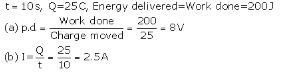Question 26:
(a) Define electric current. What is the SI unit of electric current.
(b) One coulomb of charge flows through any cross-section of a conductor in 1 second. What is the current flowing through the conductor ?
(c) Which instrument is used to measure electric current ? How should it be connected in a circuit ?
(d) What is the conventional direction of the flow of electric current ? How does it differ from the direction of flow of electrons ?
(e) A flash of lightning carries 10 C of charge which flows for 0.01 s. What is the current ? If the voltage is 10 MV, what is the energy ?

Solution :
(a) Electric current is the flow of electric charges (electrons) in a conductor such as a metal wire.
SI unit of electric current is ampere.
(b) 1 ampere.
(c) An ammeter is used to measure electric current. It should be connected in series with the circuit.
(d) Conventional direction of flow of electric current is from positive terminal of a battery to the negative terminal, through the outer circuit. The direction of flow of electrons is opposite to the direction of conventional current, i.e. from negative terminal to positive terminal.

(e) Q = 10C, t= 0.01s

I=Q/t = 10/0.01 = 1000A

p.d = W/Q

W=p.d x Q

=10 x 106 x 10 = 100 x 106 = 100MJ

Energy = work done = 100MJ

Question 32:
A student made an electric circuit shown here to measure the current through two lamps.
(a) Are the lamps in series or parallel ?
(b) The student has made a mistake in this circuit.
What is the mistake ?
(c) Draw a circuit diagram to show the correct way to connect the circuit.
Use the proper circuit symbols in your diagram.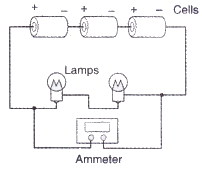Solution :
(a) Lamps are in series.
(b) Student has connected ammeter in parallel with lamps. It should be connected in series.
(c)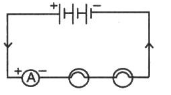Question 33:
Draw a circuit diagram to show how 3 bulbs can be lit from a battery so that 2 bulbs are controlled by the same switch while the third bulb has its own switch.
Solution :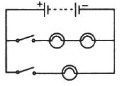Question 34:
An electric heater is connected to the 230 V mains supply. A current of 8 A flows through the heater.
(a) How much charge flows around the circuit each second ?
(b) How much energy is transferred to the heater each second ?

Solution :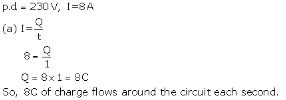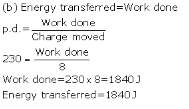Question 35:
How many electrons are flowing per second past a point in a circuit in which there is a current of 5 amp ?
Solution :

I =5A

t= 1s

I= Q/t

Q= I x t = 5 x 1 = 5c

No.  of electron comprising 1.6 x 10-13 C =1

No. of electron comprising 5C = 5/1.6 x 10-19 = 31.25 x 1018

Page No:18

Question 1:
Name the law which relates the current in a conductor to the potential difference across its ends.
Solution :
Ohm’s law

Question 2:
Name the unit of electrical resistance and give its symbol.
Solution :

Unit of electrical resistance os ohm. Its symbol is Ω.

Question 3:
Name the physical quantity whose unit is “ohm”.
Solution :
Electric resistance.

Question 4:
What is the general name of the substances having infinitely high electrical resistance ?
Solution :
Insulators.

Question 5:
Keeping the resistance constant, the potential difference applied across the ends of a component is halved. By how much does the current change ?
Solution :

V= IR

Keeping R constant, V∝I

So, when V is halved, I also become half.

Question 6:
State the factors on which the strength of electric current flowing in a given conductor depends.
Solution :
Strength of electric current flowing in a given conductor depends on
(i) potential difference across the ends of the conductor
(ii) resistance of the conductor.

Question 7:
Which has less electrical resistance : a thin wire or a thick wire (of the same length and same material) ?
Solution :
Thick wire.

Question 8:
Keeping the potential difference constant, the resistance of a circuit is halved. By how much does the current change ?
Solution :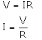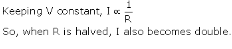Question 9:
A potential difference of 20 volts is applied across the ends of a resistance of 5 ohms. What current will flow in the resistance ?
Solution :
Potential difference, V = 20V
Resistance, R = 5ohms
Current, I = ?
We know that
V = IR
20 = I x 5
I = 20/5 = 4 A

Question 10:
A resistance of 20 ohms has a current of 2 amperes flowing in it. What potential difference is there between its ends ?
Solution :
R = 20ohms
I = 2amp
We know that
V = IR
Thus,
V = 2 x 20
V = 40V

Question 11:
A current of 5 amperes flows through a wire whose ends are at a potential difference of 3 volts. Calculate the resistance of the wire.
Solution :
I = 5amp
p.d., V = 3V
We know that
V=IR
Thus,
3 = 5 x R
R = 3/5 = 0.6 ohm

Question 12:
Fill in the following blank with a suitable word :
Ohm’s law states a relation between potential difference and……………………

Solution :
current.

Question 13:
Distinguish between good conductors, resistors and insulators. Name two good conductors, two resistors and two insulators.
Solution :
Those substances which have very low electrical resistance are called as good conductors. E.g., copper and aluminium.
Those substances which have comparatively high resistance than conductors are known as resistors. E.g., nichrome and manganin.
Those substances which have infinitely high electrical resistance are called insulators. E.g., rubber and wood.

Question 14:
Classify the following into good conductors, resistors and insulators :
Rubber, Mercury, Nichrome, Polythene, Aluminium, Wood, Manganin, Bakelite, Iron, Paper, Thermocol, Metal coin

Solution :
Conductor :- mercury, aluminum, iron, metal coin
Resistor :- manganin, nichrome
Insulator :- rubber, polythene, wood, bakelite, paper, thermocol

Offer running on EduRev: Apply code STAYHOME200 to get INR 200 off on our premium plan EduRev Infinity!

94 docs

,

,

,

,

,

,

,

,

,

,

,

,

,

,

,

,

,

,

,

,

,

,

,

,

;